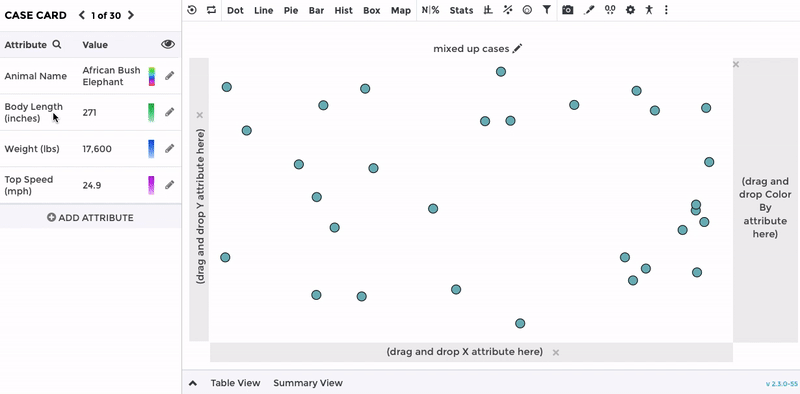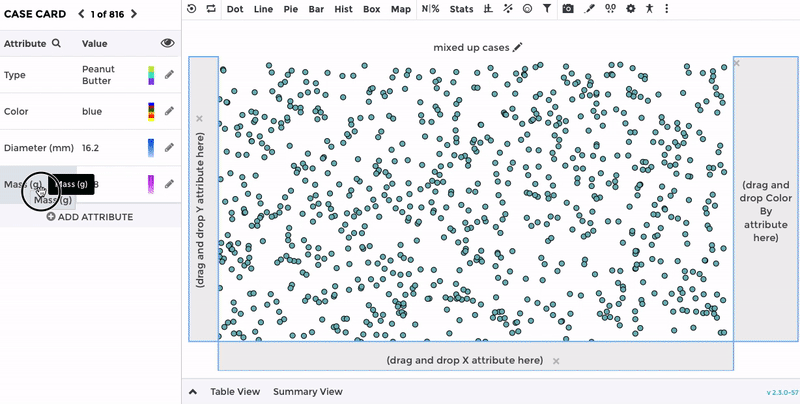# Tuva for Middle School Math

Introduction

Student in Grades 6-8 use Tuva to explore distributions of data to better understand variability and central tendency. The dynamic plotting of data allows students to visualize the relationships between dot plots, box plots, and histograms and to understand the impact of outliers on measures of center and spread.

Students also construct bivariate plots to analyze patterns of association between two quantities and begin to create functions to model those relationships. Students are engaged by both the visual aspects of Tuva as well as the many different contexts of the datasets that allow them to contextualize the math concepts that they are learning.

Measures of Center - Mean and Median

Tuva allows middle school students to visualize challenging new concepts like how the mean and median are affected by extreme values in a data set and the impact of removing those data points. Students can quickly change between a dot plot and box plot to see how the measures of center are represented differently on each graph, allowing students to build more connections about these concepts.Students can better compare distributions to visually assess for overlap and distribution shapes. The dynamic visualization helps middle school students to describe distribution shape, center, and spread when working with different graph types. Students can then use the context of the dataset to better understand why the distributions would have different centers and spread.Tuva is a powerful tool for helping students explore patterns of association in bivariate data for the first time. Students can quick drag and drop attributes to see what types of relationships exist between variables. As students begin to explore modeling linear relationships, Tuva provides multiple entry points for students such as using their own moveable line to try to best fit the data.